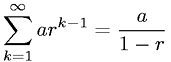Equations > Calculus > Sequences and Series > Infinite Geometric Series

### Infinite Geometric SeriesLatex Code:

MathML Code:

 $\sum _{k=1}^{\infty }{\mathrm{ar}}^{k-1}=\fraca1-r$

MathType 5.0: